# Chapter 3 Pair of Linear Equation in Two Variables NCERT Exemplar Solutions Exercise 3.1 Class 10 Maths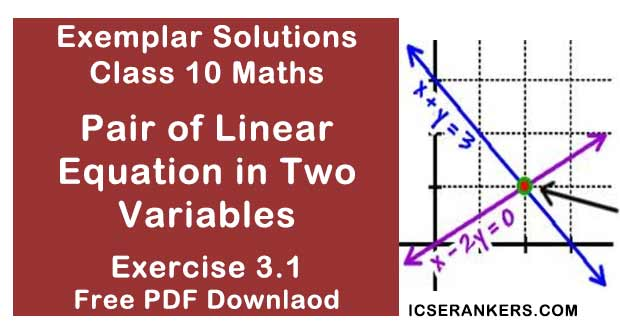Chapter Name NCERT Maths Exemplar Solutions for Chapter 3 Pair of Linear Equation in Two Variables Exercise 3.1 Book Name NCERT Exemplar for Class 10 Maths Other Exercises Exercise 3.2Exercise 3.3Exercise 3.4 Related Study NCERT Solutions for Class 10 Maths

### Exercise 3.1 Solutions

Choose the correct answer from the given four options in the following questions:

1. Graphically, the pair of equations
6x – 3y + 10 = 0
2x – y + 9 = 0 ;
Represents two lines which are:

(a) intersecting at exactly one point
(b) intersecting at exactly two points
(c) coincident at exactly two points
(d) parallel

Solution

(d)
a1 = 6
b1 = - 3
c1 = 10
a2 = 2
b2 = -1
c2 = 9
On solving, we get,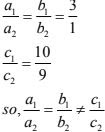So, the linear equation has no solution and the two lines are parallel.

2. The pair of equations x + 2y + 5 = 0 and –3x – 6y + 1 = 0 have
(a) a unique solution
(b) exactly two solution
(c) infinitely many solutions
(d) no solution

Solution

On solving we get,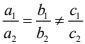Therefore, the pair of equations has no solution.

3. If a pair of linear equations is consistent, then the lines will be,
(a) parallel
(b) always coincident
(c) intersecting or coincident
(d) always intersecting

Solution

(c) Intersecting or coincident
For linear equations to be consistent, we have following conditions,4. The pair of equations y = 0 and y = -7 has
(a) one solution
(b) two solutions
(c) infinitely many solutions
(d) no solution

Solution

(d) no solution
The two equations above represents  a pair of parallel lines. Therefore, there is no solution.

5. The pair of equations x = a and y = b graphically represents lines which are
(a) parallel
(b) intersecting at (b, a)
(c) coincident
(d) intersecting at (a, b)

Solution

(d) Intersecting at (a,b)
x = a is a straight line parallel to the y–axis.
Again,
y = b is a straight line parallel to the x–axis.
Therefore, the equations x = a and y = b graphically represents lines which intersects at (a, b).
Hence, (d) is the correct answer.

6. For what value of k do the equations 3x - y + 8 = 0 and 6x - ky = -16 represent coincident lines ?
(a) 1/2
(b) -1/2
(c) 2
(d) -2

Solution

(c) 2
3x - y = -18 ...(i)
6x - ky = -16 ...(ii)
For coincident lines,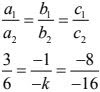On solving, we get,
k = 2.

7. If the line given by 3x + 2ky = 2 and 2x + 5y + 1 = 0 are parallel, then the value of k is
(a) -5/4
(b) 2/3
(c) 15/4
(d) 3/2

Solution

(c) 15/4
For parallel lines,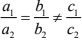On solving we get,
4k = 15
⇒ k = 15/4

8. The value of c for which the pair of equations cx – y = 2 and 6x – 2y = 3 will have infinity many solutions is
(a) 3
(b) –3
(c) –12
(d) No value

Solution

(d) no value
To have infinitely many solutions,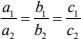On solving, we get that there are no common values of c. Hence, there is no value of c for which the equations have infinitely many solutions.

9. One equation of a pair of dependent linear equations is –5x + 7y = 2. The second equation can be:(a) 10x + 14y + 4 = 0
(b) –10x – 14y + 4 = 0
(c)–10x + 14y + 4 = 0
(d) 10x – 14y = –4

Solution

(d) 10x - 14y = - 4
-2(-5x + 7y -2) = 0
-5x + 7y - 2 = 0 ...(i)
Let the second equation be,
a2x + b2y + c2 = 0 ...(ii)
∴ For dependent system of linear equations,So,
a2 = -5k,
b2 = 7k
c2 = -2k
Putting value of k,
k = 0, and -1 it does not satisfy the required condition.
Let us take k = -2
So,
a2  = +10
b2 = -14
c2 = + 4
It satisfies the condition.

10. A pair of linear equations which has a unique solution x = 2, y = –3 is
(a) x + y = –1 and 2x – 3y = –5
(b) 2x + 5y = –11 and 4x + 10y = –22
(c) 2x – y = 1 and 3x + 2y = 0
(d) x – 4y –14 = 0 and 5x – y – 13 = 0

Solution

b and d both.
As x = 2, y = -3 is unique solution of system of  equation so these values of must satisfy both equations.

(a) x + y = -1 and 2x - 3y = - 5
Putting x = 2 and y = 3 in both the equations.
LHS = x + y
2 - 3 = -1 (RHS)
LHS = 2x – 3y
= 2(2) –3(–3)
= 4 + 9
= 13
≠ RHS

(b) 2x + 5y = –11 and 4x + 10y = –22
Put x = 2 and y = –3 in both the equations.
LHS = 2x + 5y
= 2 × 2 + (–3)
= 4 – 15
= –11
= RHS
LHS = 4x + 10y
= 4(2) + 10(–3)
= 8 – 30
= –22
= RHS

(c) 2x – y = 1 and 3x + 2y = 0
Put x = 2 and y = –3 in both the equations.
LHS = 2x – y
2(2) + 3 = 7 ≠ RHS
LHS = 3x + 2y
= 3(2) + 1(–3)
= 6 – 6
= 0
= RHS

(d) x – 4y – 14 = 0 and 5x – y – 13 = 0
x – 4y = 14 and 5x – y = 13
Put x = 2 and y = –3 in both the equations.
LHS = x – 4y
= 2 – 4(–3)
= 2 + 12
= 14 = RHS
LHS = 5x – y
= 5(2) – (–3)
= 10 + 3
= 13 = RHS
So, the pair of equations is (b) and (d).

11. If x = a, y = b, is the solution of the equations x - y = 2 and x + y = 4, then the values of a and b are, respectively
(a) 3 and 5
(b) 5 and 3
(c) 3 and 1
(d) –1 and –3
Solution
(c)
If (a, b) is the solution of the given equations, then it must satisfy the given equations so,
a – b = 2 ...(i)
a + b = 4 ...(ii)
2a = 6 [solving (i) and (ii)]
⇒ a = 3
Now,
3 + b = 4 [From (ii)]
⇒ b = 1
So,
(a, b) = (3, 1).

12. Aruna has only ₹1 and ₹2 coins with her. If the total number of coins that she has is50 and the amount of money with her is ₹75, then the number of ₹1 and ₹2 coins are respectively:
(a) 35 and 15
(b) 35 and 20
(c) 15 and 35
(d) 25 and 25
Solution
(d)
Let the number of ₹1 coins = x
and the number of ₹2 coins = y
So,
x+y = 50 ...(i)
1x+2y = 75 ...(ii)
On subtracting equation (i)from (ii)
y = 25
Putting value of y in (i)
x=25
So,
y = 25 and x = 25.

13. The father’s age is six times his son, age. Four years hence, the age of the father willbe four times his son’s age. The present ages, (in years) of the son and the father are,respectively.
(a) 4 and 24
(b) 5 and 30
(c) 6 and 36
(d) 3 and 24
Solution
(c)
Let,
Present age of father be x years
and
Present age of son be y years.
According to the question,
x = 6y ...(i)
Age of the father after four years = (x + 4) years
Similarly,
Age of son after four years = (y + 4) years
Now,
x + 4 = 4(y + 4) ...(ii)
⇒ x + 4 = 4y + 16
⇒ 6y – 4y = 16 – 4 [as, x = 6y]
⇒ 2y = 12
⇒ y = 6
So,
x = 6 × 6
⇒ x = 36 years [From (i)]
Therefore, the present ages of the son and the father are 6 years and 36 years respectively.